# A sticky shortcut

Last week, Dan Moren wrote a nice post about calculating the heat index in Shortcuts. I’d written a similar shortcut a few days earlier to calculate the dewpoint (it must be a summer thing), but it was kind of crappy and I had put it aside. Inspired by Dan’s work, I returned to my dewpoint shortcut and cleaned it up.

While I’m sure the dewpoint is included in some weather apps, it isn’t in the stock Weather app. It is, in theory at least, part of the data set returned by the action in Shortcuts. I say “in theory” because I’ve found that quite often the Dewpoint entry is empty when that action is run. Why that is I don’t know, but that was the reason my original dewpoint shortcut was disappointing.

The improved shortcut, which you can download, uses a calculation when falls short. Here it is:

StepActionComment
1This puts all the weather data into the magic variable Weather Conditions
2This checks whether the dewpoint is included in Weather Conditions. You might think the condition should be “has any value,” but that didn’t work when I tried it.
3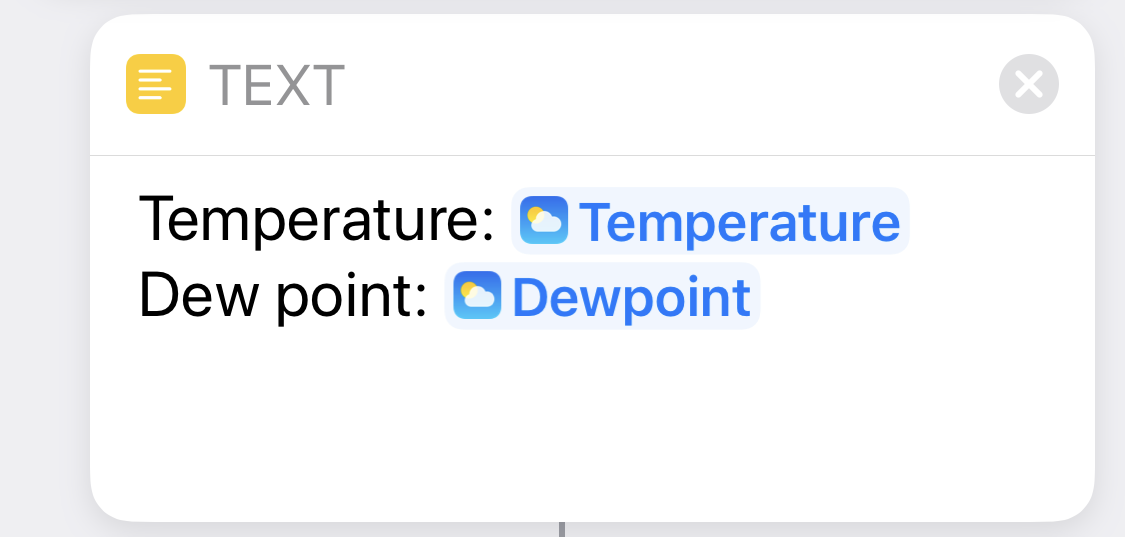If there is a Dewpoint value, use it in the output text.
45If there isn’t a Dewpoint value…
6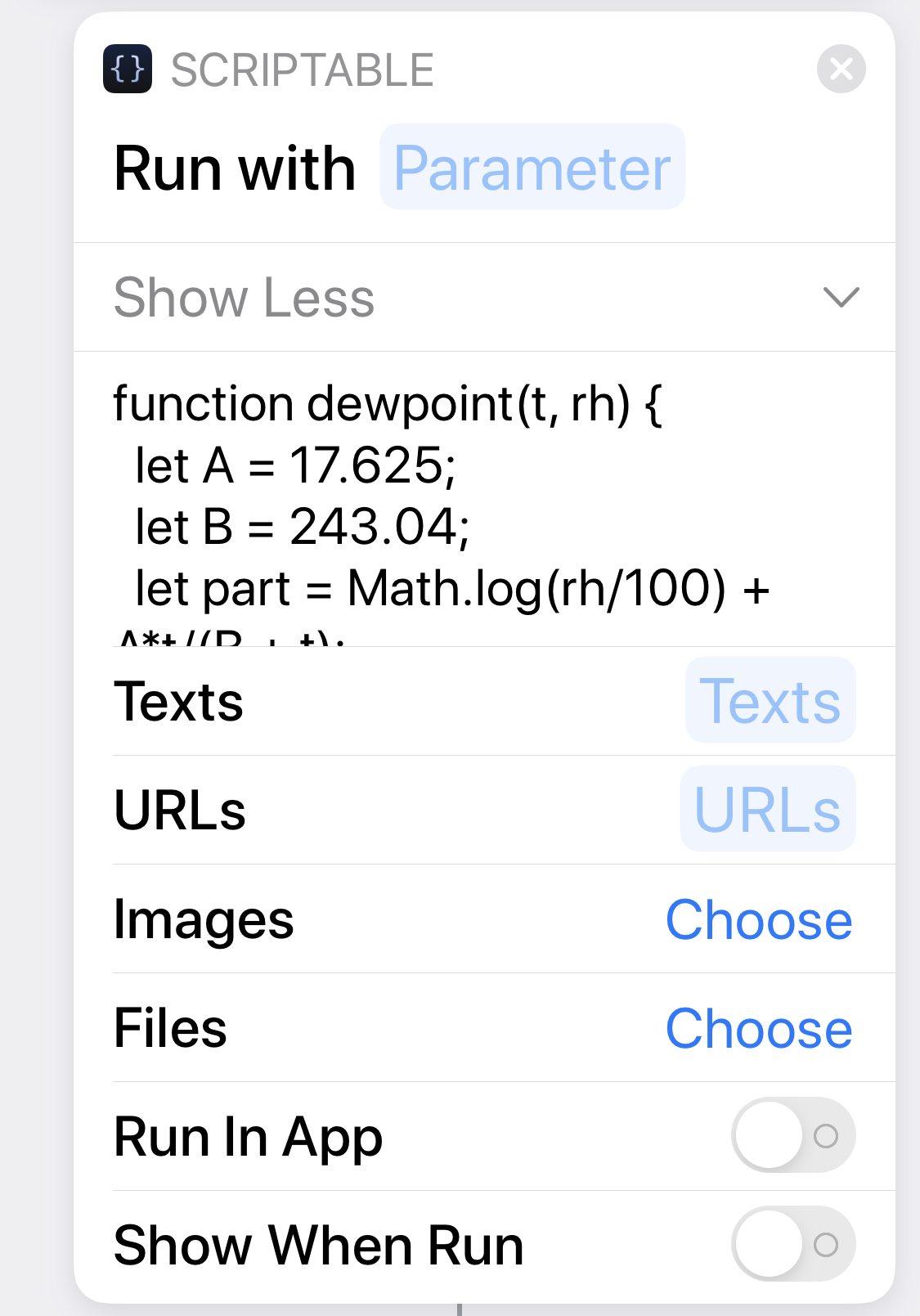Calculate the dewpoint from the temperature and relative humidity. The full code for this step is below.
7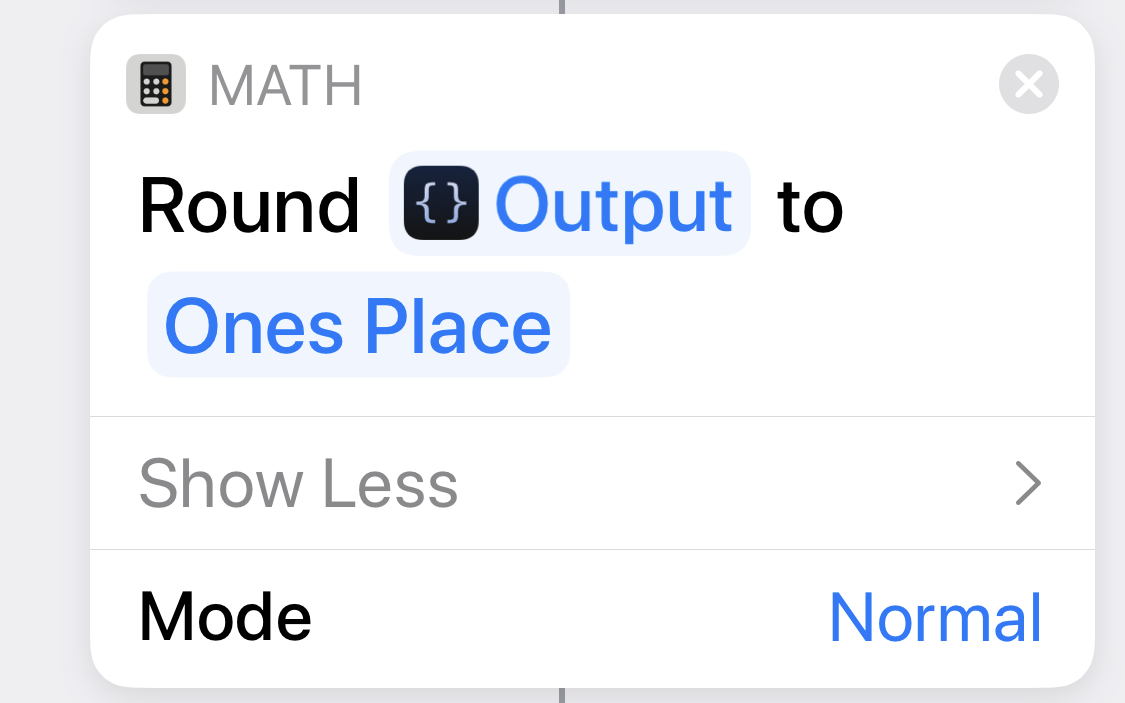Round the output to the nearest whole number. I could have done this in the Scriptable step, but it was easy enough to do in Shortcuts directly
8Build the output from the calculated value.
910The Weather Conditions magic variable is used repeatedly in the shortcut to get at certain data. For example, when we need the temperature, we add Weather Conditions and choose Temperature from the list of data.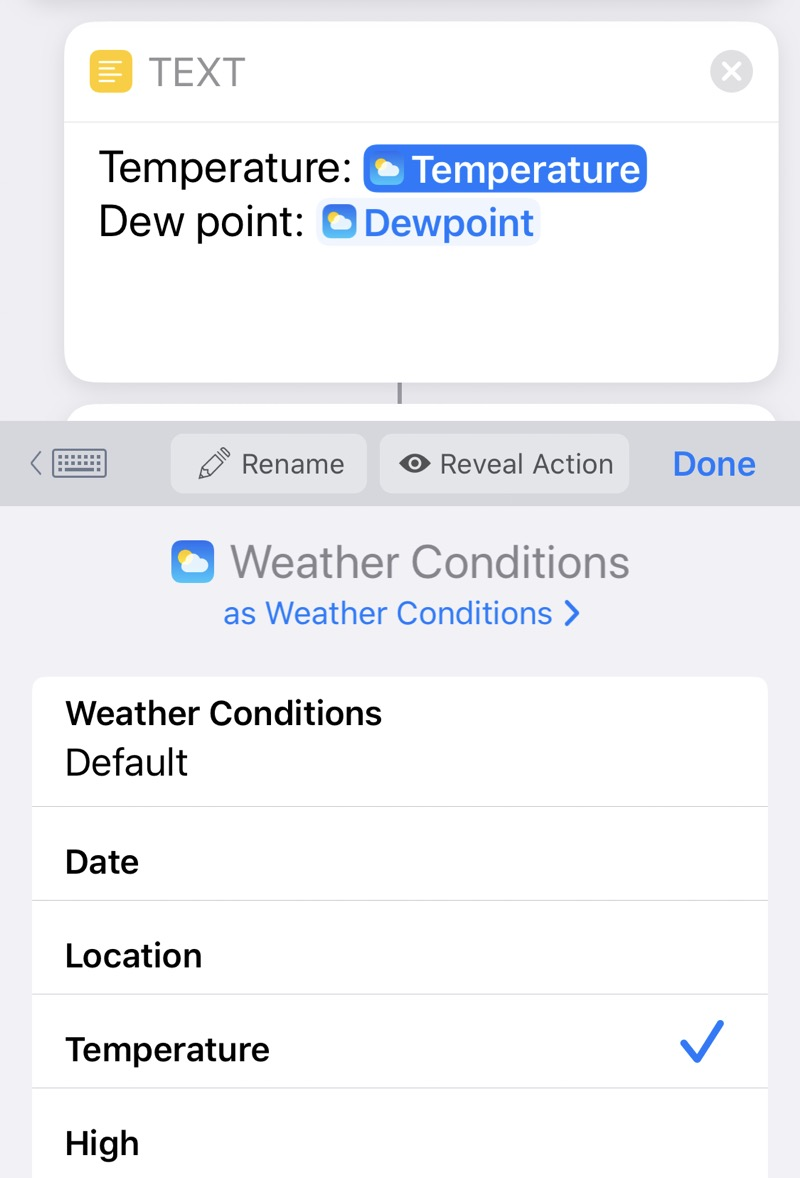As you can see, the name of the type of data chosen is displayed in the shortcut. For certain data, like Temperature, we also get to choose the units.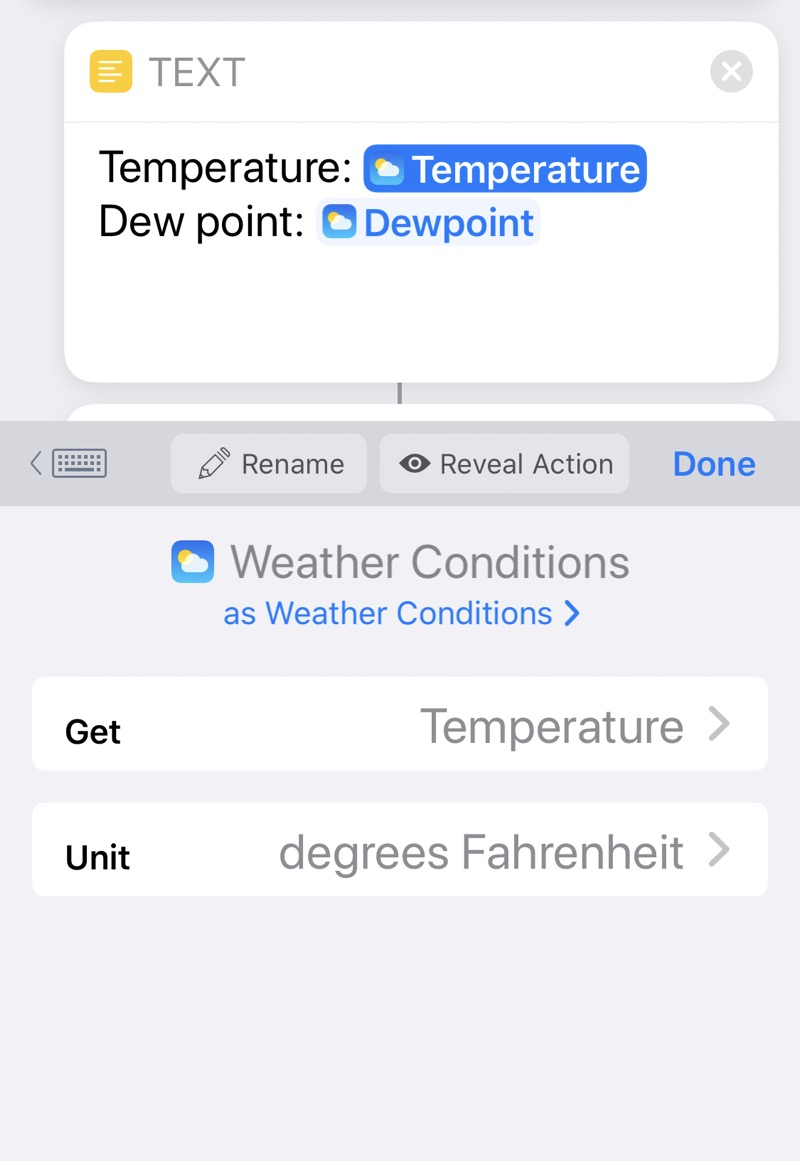Here’s the JavaScript code from Step 6:

javascript:
1:  function dewpoint(t, rh) {
2:    let A = 17.625;
3:    let B = 243.04;
4:    let part = Math.log(rh/100) + A*t/(B + t);
5:    return B*part/(A - part);
6:  }
7:
8:  let rh = ☐ Weather Conditions (Humidity);  // number
9:  let t = '☐ Weather Conditions (Temperature)'; // string
10:  t = t.match(/[-\d.]+/);
11:  t = parseFloat(t);
12:
13:  return dewpoint(t, rh)*9/5 + 32;


The dewpoint function is based on this equation:

$T_d = \frac{ B \left[ \ln \left( \frac{RH}{100} \right) + A \frac{T}{B + T} \right]}{A - \left[ \ln \left( \frac{RH}{100} \right) + A \frac{T}{B + T} \right] }$

This is a curve fit, where $$T$$ is the normal (dry bulb) temperature in Celsius, $$RH$$ is the relative humidity in percent, and $$T_d$$ is the dewpoint in Celsius. The parameters that give a good fit are $$A = 17.625$$ and $$B = 243.04$$. I got the formula from this nice survey paper by Mark Lawrence. Unfortunately, the paper has a typo in the value for $$A$$, and I had to look up the original paper by Oleg Alduchov and Robert Eskridge to get the correct value. Scholarship!

One of the great things about doing inline Scriptable is that you can stick Shortcuts variables directly into your JavaScript. That’s what’s happening with those weird-looking parts of Lines 8 and 9. Within the Scriptable step itself, they look like this: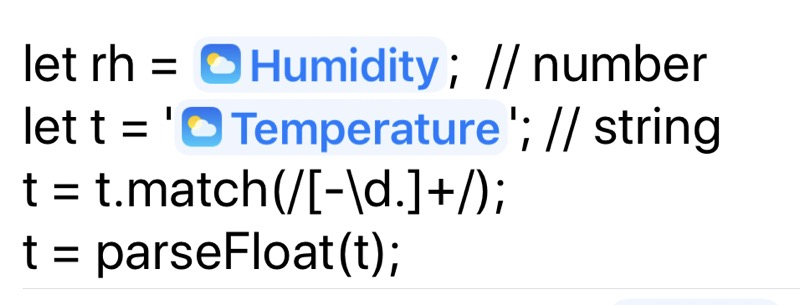While the humidity is given as a number, the temperature is given as a string with “°C” at the end. Lines 10–11 extract the numeric part from the string1 and convert it from a string to a floating point number.

Finally, Line 13 converts the dewpoint temperature from Celsius to Fahrenheit. Any complaints about this will be ignored.

Running Dewpoint directly from Shortcuts returns the output in this form:Running it in the Shortcuts widget on my phone returns the output within the widget: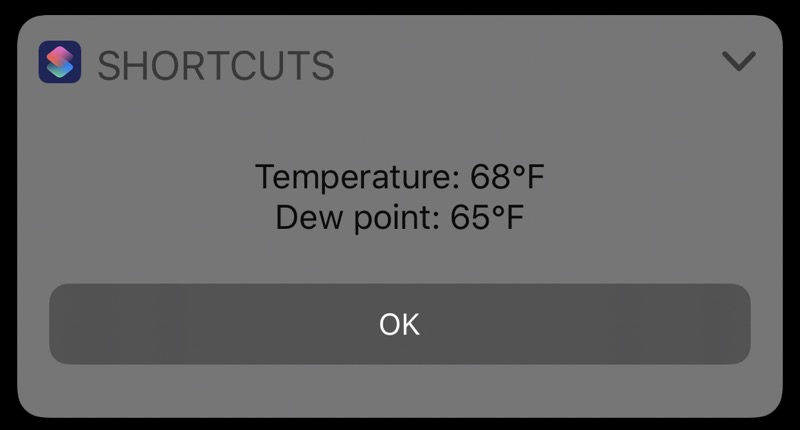This output is fine as written but not so good when spoken by Siri. There’s no pause between the temperature and the dewpoint. If experience shows that I’ll be using it mostly through Siri, I’ll change the Text definitions to be more like sentences.

1. No, I didn’t use the complex regex from my earlier post because I knew this simpler form would work. Also, I wrote this script before I came up with the more complex regex.